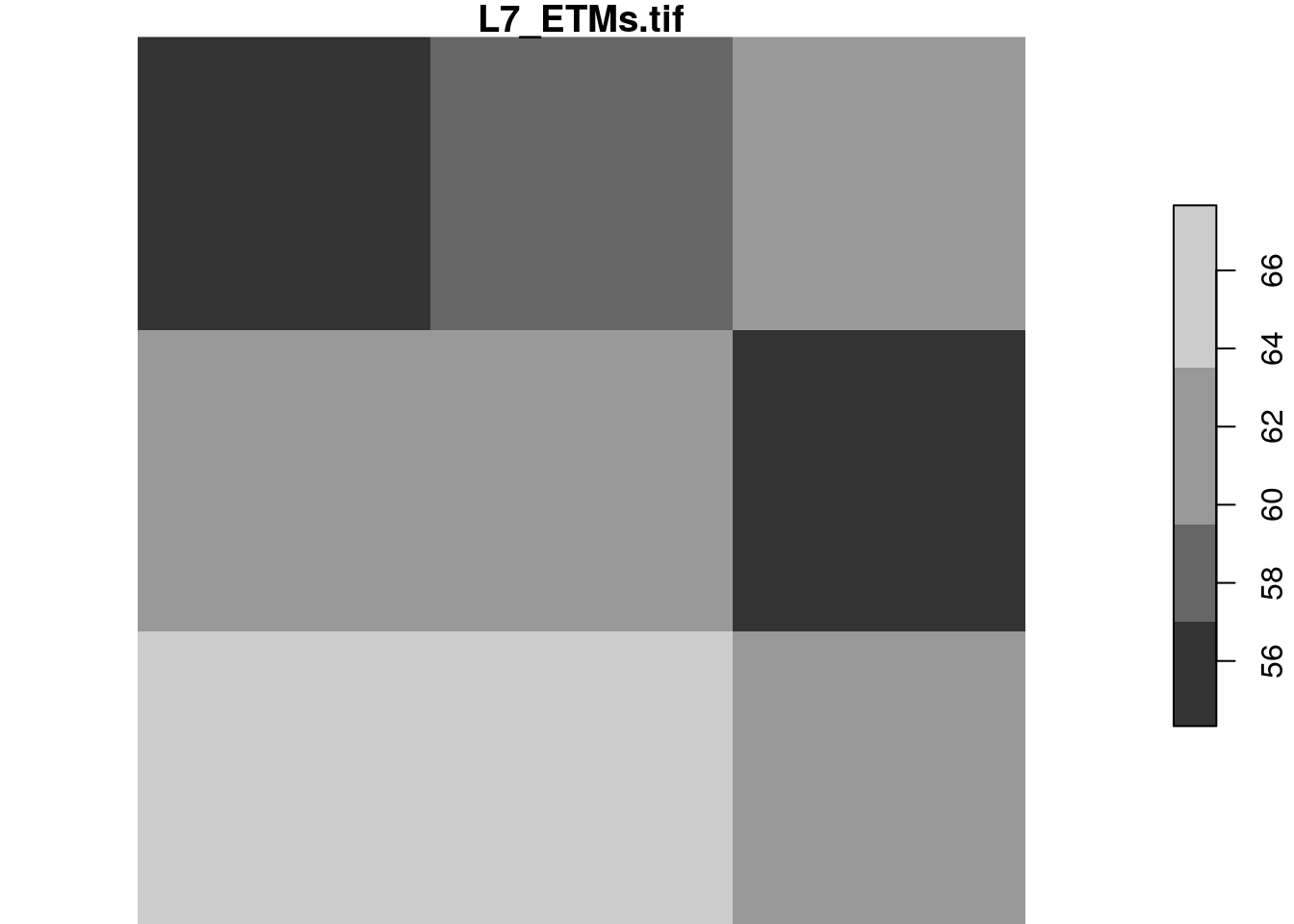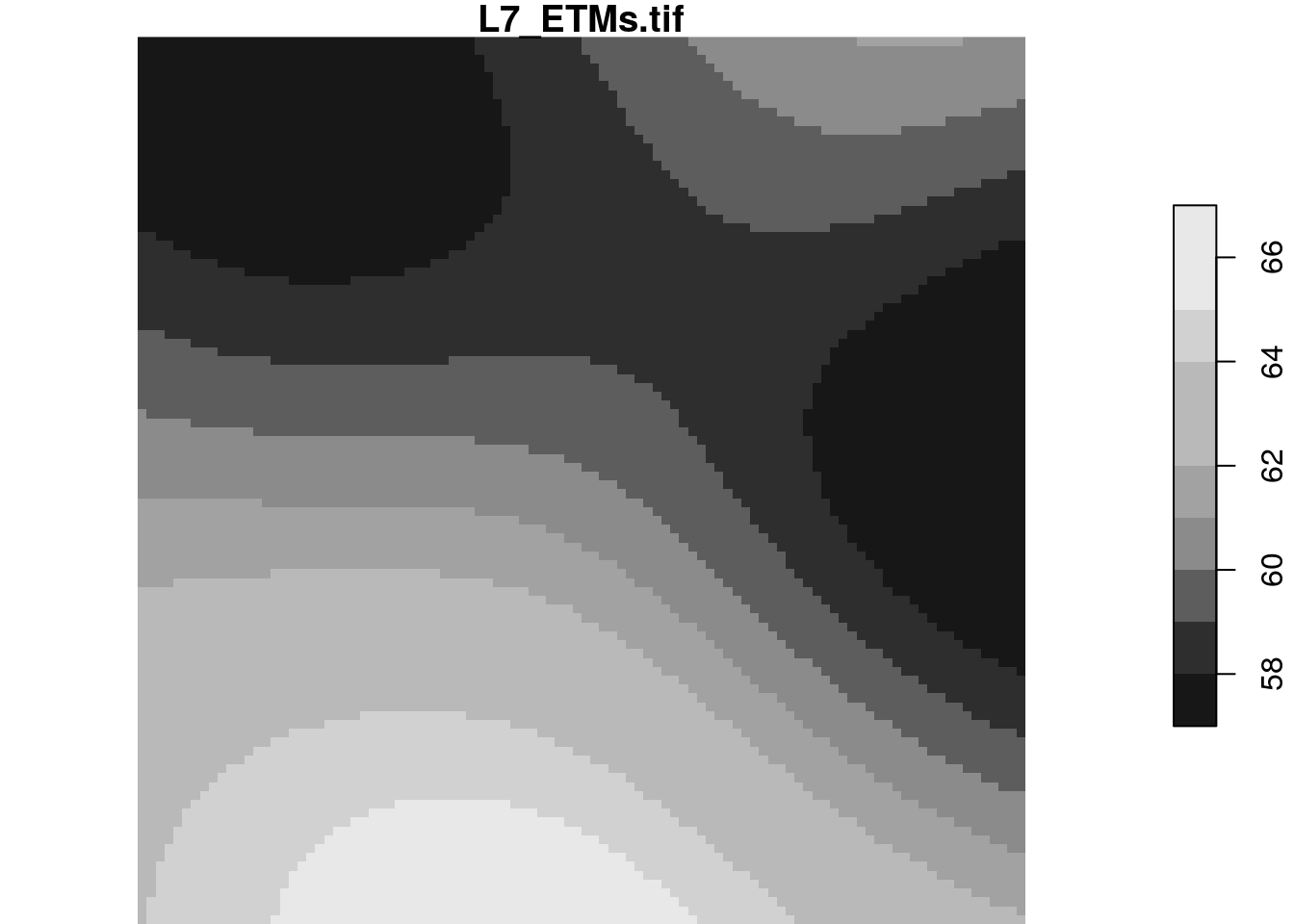When your imagery or array data easily fits a couple of times in R’s working memory (RAM), consider yourself lucky. This document was not written for you. If your imagery is too large, or for other reasons you want to work with smaller chunks of data than the files in which they come, read on about your options. First we will discuss the low-level interface for this, then the higher level, using stars proxy objects that delay all reading.

# Preamble: the starsdata package

To run all of the examples in in this vignette, you must install a package with datasets that are too large (1 Gb) to be held in the `stars` package. They are in a drat repo, installation is done by

``install.packages("starsdata", repos = "http://pebesma.staff.ifgi.de", type = "source") ``

# Reading chunks, change resolution, select bands

`read_stars` has an argument called `RasterIO` which controls how a GDAL dataset is being read. By default, all pixels and all bands are read in memory. This can consume a lot of time and require a lot of memory. Remember that your file may be compressed, and that pixel values represented in the file by bytes are converted to 8-byte doubles in R.

The reason of using `RasterIO` for this is that the parameters we use are directly mapped to the GDAL RasterIO function used (after adapting the 1-based offset index in R to 0-based offset in C++).

We can read a (spatially) rectangular chunk of data by

An example of using `RasterIO` is

``````library(stars)
tif = system.file("tif/L7_ETMs.tif", package = "stars")
rasterio = list(nXOff = 6, nYOff = 6, nXSize = 100, nYSize = 100, bands = c(1,3,4))
(x = read_stars(tif, RasterIO = rasterio))
## stars object with 3 dimensions and 1 attribute
## attribute(s):
##   L7_ETMs.tif
##  Min.   : 23.00
##  1st Qu.: 54.00
##  Median : 63.00
##  Mean   : 62.06
##  3rd Qu.: 73.25
##  Max.   :235.00
## dimension(s):
##      from  to  offset delta                       refsys point values
## x       6 105  288776  28.5 +proj=utm +zone=25 +south... FALSE   NULL [x]
## y       6 105 9120761 -28.5 +proj=utm +zone=25 +south... FALSE   NULL [y]
## band    1   3      NA    NA                           NA    NA   NULL
dim(x)
##    x    y band
##  100  100    3``````

Compare this to

``````st_dimensions(read_stars(tif))
##      from  to  offset delta                       refsys point values
## x       1 349  288776  28.5 +proj=utm +zone=25 +south... FALSE   NULL [x]
## y       1 352 9120761 -28.5 +proj=utm +zone=25 +south... FALSE   NULL [y]
## band    1   6      NA    NA                           NA    NA   NULL``````

and we see that

• the `delta` values remain the same,
• the offset (x/y coordinates of origing) of the grid remain the same
• the `from` and `to` reflect the new area, and relate to the new `delta` values
• `dim(x)` reflects the new size, and
• only three bands were read

## Reading at a different resolution

Reading datasets at a lower (but also higher!) resolution can be done by setting `nBufXSize` and `nBufYSize`

``````rasterio = list(nXOff = 6, nYOff = 6, nXSize = 100, nYSize = 100,
nBufXSize = 20, nBufYSize = 20, bands = c(1,3,4))
(x = read_stars(tif, RasterIO = rasterio))
## stars object with 3 dimensions and 1 attribute
## attribute(s):
##   L7_ETMs.tif
##  Min.   : 29.00
##  1st Qu.: 54.00
##  Median : 64.00
##  Mean   : 62.28
##  3rd Qu.: 73.00
##  Max.   :107.00
## dimension(s):
##      from to  offset  delta                       refsys point values
## x       2 21  288776  142.5 +proj=utm +zone=25 +south... FALSE   NULL [x]
## y       2 21 9120761 -142.5 +proj=utm +zone=25 +south... FALSE   NULL [y]
## band    1  3      NA     NA                           NA    NA   NULL``````

and we see that in addition:

• the `delta` (raster cell size) values have increased a factor 5, because `nBufXSize` and `nBufYSize` were set to values a factor 5 smaller than `nXSize` and `nYSize`
• the offset coordinates of the grid are still the same
• the `from` and `to` reflect the new area, but relate to the new `delta` cell size values

We can also read at higher resolution; here we read a 3 x 3 area and blow it up to 20 x 20:

``````rasterio = list(nXOff = 6, nYOff = 6, nXSize = 3, nYSize = 3,
nBufXSize = 100, nBufYSize = 100, bands = 1)
x = read_stars(tif, RasterIO = rasterio)
dim(x)
##   x   y
## 100 100
plot(x)``````The reason we “see”" only three grid cells is that the default sampling method is “nearest neighbour”. We can modify this by

``````rasterio = list(nXOff = 6, nYOff = 6, nXSize = 3, nYSize = 3,
nBufXSize = 100, nBufYSize = 100, bands = 1, resample = "cubic_spline")
x = read_stars(tif, RasterIO = rasterio)
dim(x)
##   x   y
## 100 100
plot(x)``````The following methods are allowed for parameter `resample`:

`resample` method used
`nearest_neighbour` Nearest neighbour (default)
`bilinear` Bilinear (2x2 kernel)
`cubic` Cubic Convolution Approximation (4x4 kernel)
`cubic_spline` Cubic B-Spline Approximation (4x4 kernel)
`lanczos` Lanczos windowed sinc interpolation (6x6 kernel)
`average` Average
`mode` Mode (selects the value which appears most often of all the sampled points)
`Gauss` Gauss blurring

All these methods are implemented in GDAL; for what these methods exactly do, we refer to the GDAL documentation or source code.

# Stars proxy objects

Stars proxy objects take another approach: upon creation they contain no data at all, but only pointers to where the data can be read. Data is only read when it is needed, and only as much as is needed: if we plot a proxy objects, the data are read at the resolution of pixels on the screen, rather than at the native resolution, so that if we have e.g. a 10000 x 10000 Sentinel 2 (level 1C) image, we can open it by

``````granule = system.file("sentinel/S2A_MSIL1C_20180220T105051_N0206_R051_T32ULE_20180221T134037.zip", package = "starsdata")
s2 = paste0("SENTINEL2_L1C:/vsizip/", granule, "/S2A_MSIL1C_20180220T105051_N0206_R051_T32ULE_20180221T134037.SAFE/MTD_MSIL1C.xml:10m:EPSG_32632")
(p = read_stars(s2, proxy = TRUE))
## stars_proxy object with 1 attribute in file:
## \$`MTD_MSIL1C.xml:10m:EPSG_32632`
##  "SENTINEL2_L1C:/vsizip//home/edzer/R/x86_64-pc-linux-gnu-library/3.5/starsdata/sentinel/S2A_MSIL1C_20180220T105051_N0206_R051_T32ULE_20180221T134037.zip/S2A_MSIL1C_20180220T105051_N0206_R051_T32ULE_20180221T134037.SAFE/MTD_MSIL1C.xml:10m:EPSG_32632"
##
## dimension(s):
##      from    to offset delta                       refsys point values
## x       1 10980  3e+05    10 +proj=utm +zone=32 +datum...    NA   NULL [x]
## y       1 10980  6e+06   -10 +proj=utm +zone=32 +datum...    NA   NULL [y]
## band    1     4     NA    NA                           NA    NA   NULL``````

and this happens instantly, because no data is read. When we plot this object,

``system.time(plot(p))``